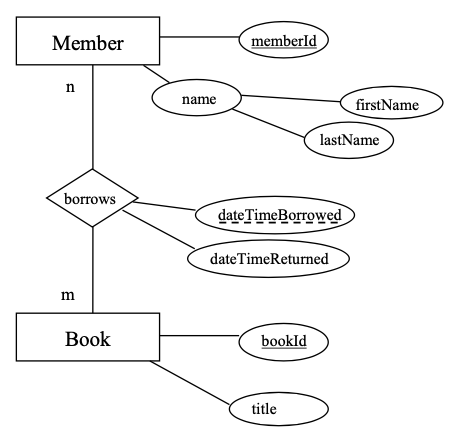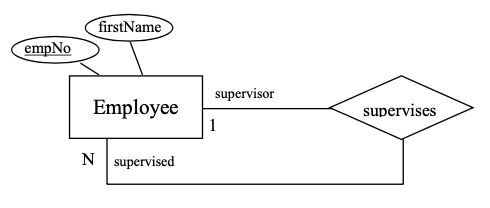# 10.2.1.5.2: Examples

•• Professor (Applied Computer Science) at University of Winnipeg

Example $$\PageIndex{1}$$

Consider the ERDFigure $$\PageIndex{1}$$: Example ERD #1.

The mapping rules lead to the relations:

Table $$\PageIndex{1}$$: Book relations.

Book

bookId

title

Table $$\PageIndex{2}$$: Member relations.

Member

memberId

firstName

lastName

Table $$\PageIndex{3}$$: Borrow relations.

Borrow

memberId

bookId

dateTimeBorrowed

dateTimeReturned

Notes:

• The Member relation does not have a composite attribute name.
• Since Borrows is a many-to-many relationship the Borrow relation is defined with a composite primary key {memberId, bookId, dateTimeBorrowed}.
• memberId in the Borrow relation is a foreign key referencing Member.
• bookId in the Borrow relation is a foreign key referencing Book.

Example $$\PageIndex{2}$$

Consider the ERDFigure $$\PageIndex{2}$$: Example ERD #2.

The mapping rules lead to the relation:

Table $$\PageIndex{4}$$: Employee relations.

Employee

empNo

firstName

supervisor

Notes:

• The attribute supervisor is a foreign key referencing Employee.
• A foreign key is placed on the ‘many’ side of a relationship and so in this case the foreign key references the employee who is the supervisor (the role name on the ‘one’ side); hence the name supervisor was chosen as the attribute name.

Example $$\PageIndex{3}$$

Consider the ERDFigure $$\PageIndex{3}$$: Example ERD #3.

The mapping rules lead to the relations.

Table $$\PageIndex{5}$$: Department relations.

Department

deptCode

deptName

chair

Table $$\PageIndex{6}$$: Course relations.

Course

deptCode

courseNo

title

creditHours

Notes:

• deptCode was chosen as the primary key of Department.
• deptName is a key and so a unique index can be defined to ensure uniqueness.
• Since Course is a weak entity type and is involved in an identifying relationship, the primary key of Course is composite comprising {deptCode, courseNo}.
• deptCode in Course is a foreign key referencing Department.

## Exercises

Exercise $$\PageIndex{1}$$

Map the ERD to relations.

Exercise $$\PageIndex{2}$$

Map the ERD to relations.

Exercise $$\PageIndex{3}$$

Map the ERD to relations.